# 4.1   Analysis of Algorithms

In this section, you will learn to respect a principle whenever you program: Pay attention to the cost. To study the cost of running them, we study our programs themselves via the scientific method. We also apply mathematical analysis to derive concise models of the cost.

## Scientific method.

The following five-step method summarizes the scientific method: the following 5 step approach.
• Observe some feature of the natural world.
• Hypothesize a model that is consistent with the observations.
• Predict events using the hypothesis.
• Verify the predictions by making further observations.
• Validate by repeating until the hypothesis and observations agree.
The experiments we design must be reproducible and the hypotheses we formulate must be falsifiable.

## Observations.

Measuring the exact running time of our program is difficult, but there are a number of tools available to help. In this book, we simply run a program on various inputs and measure the amount of time to process each input using the Stopwatch.java data type. Our first qualitative observation about most programs is that there is a problem size that characterizes the difficulty of the computational task. Normally, the problem size is either the size of the input or the value of a command-line argument. Intuitively, the running time should increase with the problem size, but the question of how much it increases naturally arises every time we develop and run a program.

## A concrete example.

To illustrate the approach, we start with ThreeSum.java which counts the number of triples in an array of $$n$$ integers that sums to 0. What is the relationship between the problem size $$n$$ and running time for ThreeSum?

• Doubling hypothesis. For a great many programs, we can quickly formulate a hypothesis for the following question: What is the effect on the running time of doubling the size of the input?
• Empirical analysis. One simple way to develop a doubling hypothesis is to double the size of the input and observe the effect on the running time. DoublingTest.java generates a sequence of random input arrays for ThreeSum, doubling the array length at each step, and prints the ratio of running times of ThreeSum.count() for each input over the previous (which was one-half the size). If you run this program, you will find that the elapsed time increases by about a factor of 8 to print each line. This leads immediately to the hypothesis that the running time increases by a factor of 8 when the input size doubles.
• Log–logplot. We might also plot the running times on a standard plot (left) or log–log plot (right). The log–log plot is a straight line with slope 3, clearly suggesting the hypothesis that the running time satisfies a power law of the form $$cn^3$$.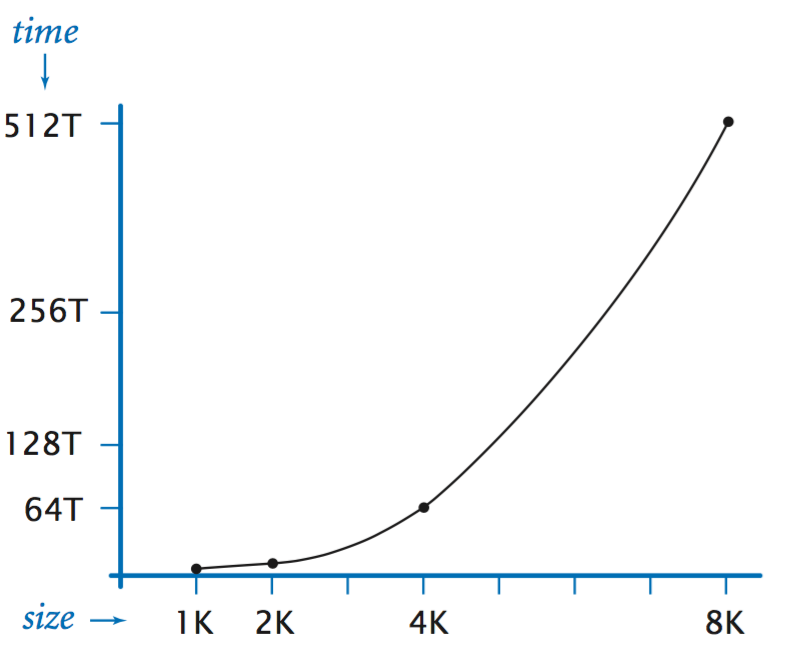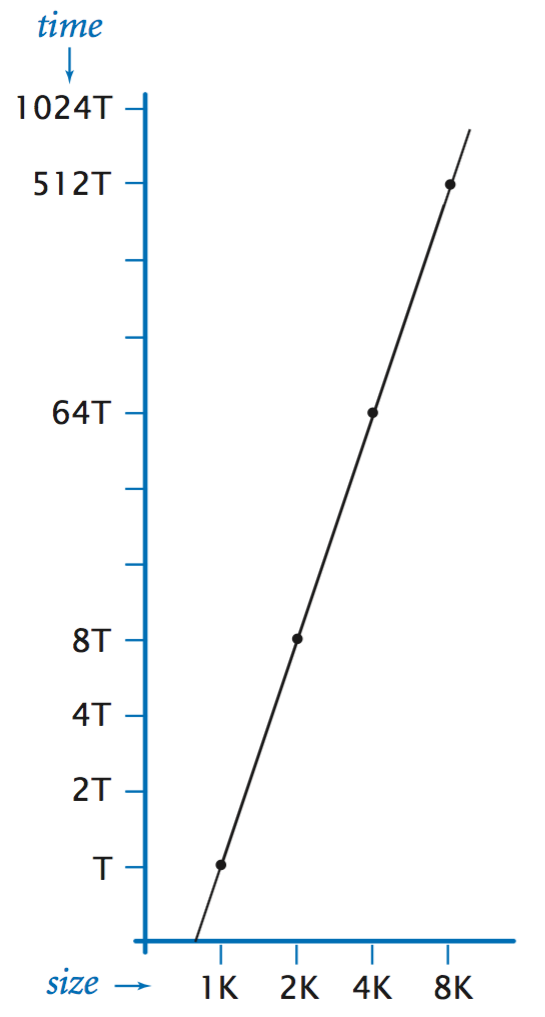• Mathematical analysis. The total running time is determined by two primary factors:
• The cost of executing each statement.
• The frequency of execution of each statement.

The former is a property of the system, and the latter is a property of the algorithm. If we know both for all instructions in the program, we can multiply them together and sum for all instructions in the program to get the running time.

The primary challenge is to determine the frequency of execution of the statements. Some statements are easy to analyze: for example, the statement that sets count to 0 in ThreeSum.count() is executed only once. Others require higher-level reasoning: for example, the if statement in ThreeSum.count() is executed precisely $$n(n-1)(n-2) / 6$$ times.

## Tilde notation.

We use tilde notation to develop simpler approximate expressions. First, we work with the leading term of mathematical expressions by using a mathematical device known as the tilde notation. We write $$\sim g(n)$$ to represent any quantity that, when divided by $$f(n)$$, approaches $$1$$ as $$n$$ grows. We also write $$g(n) \sim f(n)$$ to indicate that $$g(n) \,/\, f(n)$$ approaches $$1$$ as $$n$$ grows. With this notation, we can ignore complicated parts of an expression that represent small values. For example, the if statement in ThreeSum.count() is executed $$\sim n^3 / 6$$ times because $$n(n-1)(n-2) / 6 = n^3/6 - n^2/2 + n/3$$, which, when divided by $$n^3/6$$, approaches $$1$$ as $$n$$ grows.

We focus on the instructions that are executed most frequently, sometimes referred to as the inner loop of the program. In this program it is reasonable to assume that the time devoted to the instructions outside the inner loop is relatively insignificant.

## Order of growth.

The key point in analyzing the running time of a program is this: for a great many programs, the running time satisfies the relationship $$T(n) \sim c f(n)$$ where $$c$$ is a constant and $$f(n)$$ a function known as the order of growth of the running time. For typical programs, $$f(n)$$ is a function such as $$\log_2 n, n, n \log_2 n, n^2,$$ or $$n^3$$.

The order of growth of the running time of ThreeSum.count() is $$n^3$$. The value of the constant $$c$$ depends both on the cost of executing instructions and on details of the frequency analysis, but we normally do not need to work out the value. Knowing the order of growth typically leads immediately to a doubling hypothesis. In the case of ThreeSum.count(), knowing that the order of growth is $$n^3$$ tells us to expect the running time to increase by a factor of 8 when we double the size of the problem because

$$\lim_{n\to\infty} \frac{T(2n)}{T(n)} \;=\; \frac{c (2n)^3}{c (n)^3} \;=\; 8$$

## Order of growth classifications.

We use just a few structural primitives (statements, conditionals, loops, and method calls) to build Java programs, so very often the order of growth of our programs is one of just a few functions of the problem size, summarized in the table below.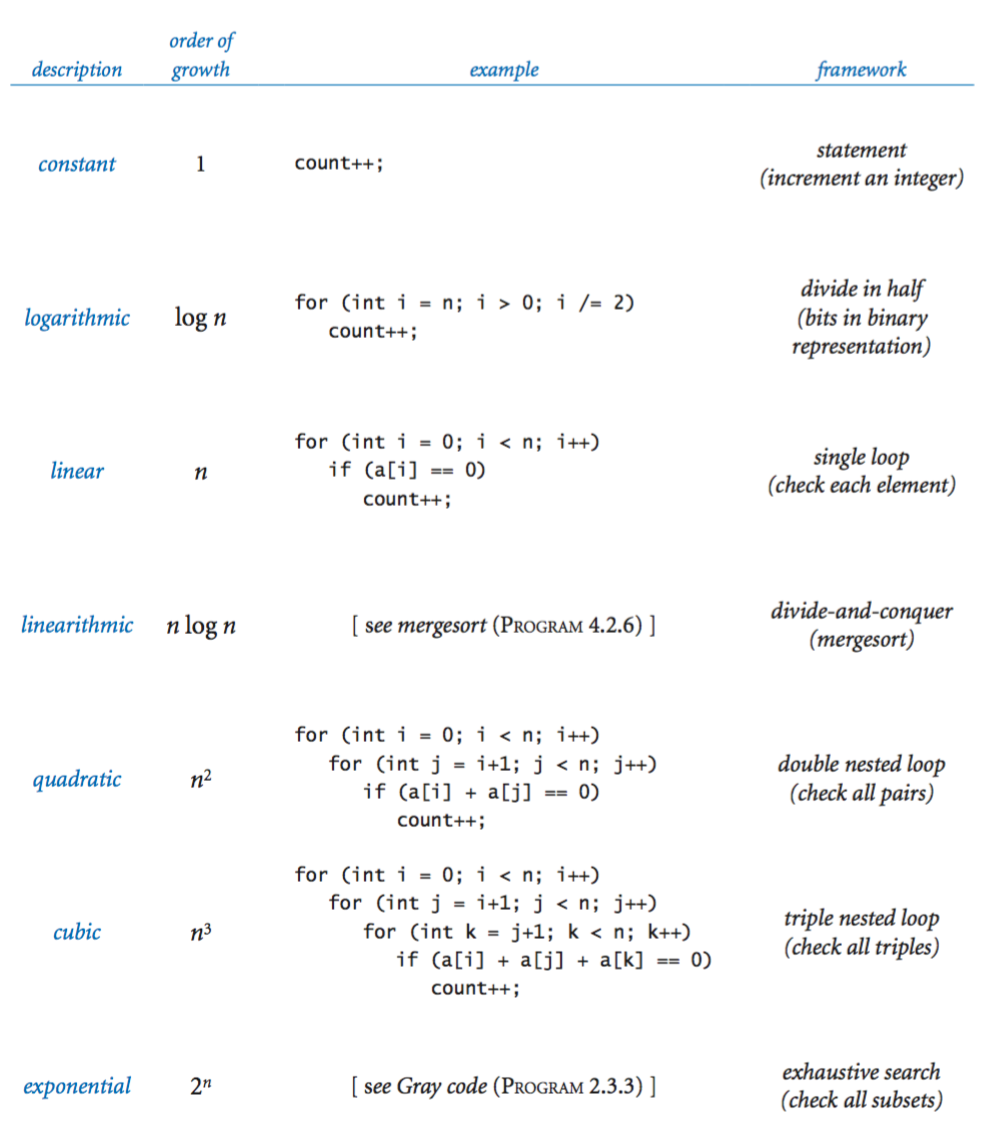## Estimating memory usage.

To pay attention to the cost, you need to be aware of memory usage. Memory usage is well-defined for Java on your computer (every value will require precisely the same amount of memory each time that you run your program), but Java is implemented on a very wide range of computational devices, and memory consumption is implementation-dependent.
• Primitive types. For example, since the Java int data type is the set of integer values between −2,147,483,648 and 2,147,483,647, a grand total of 232 different values, it is reasonable to expect implementations to use 32 bits to represent int values.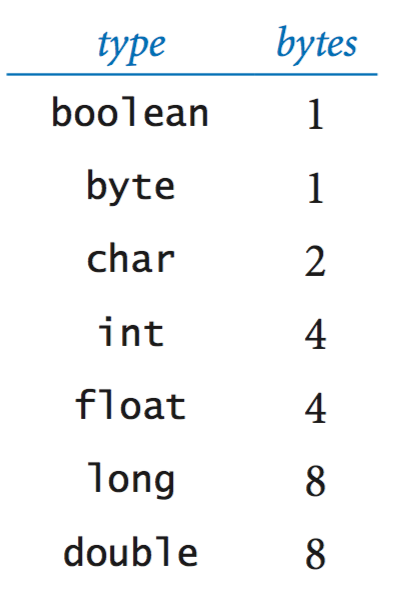• Objects. To determine the memory consumption of an object, we add the amount of memory used by each instance variable to the overhead associated with each object, typically 8 bytes. For example, a Complex.java object uses 32 bytes (16 bytes of overhead, plus 8 bytes for each of its two double instance variables).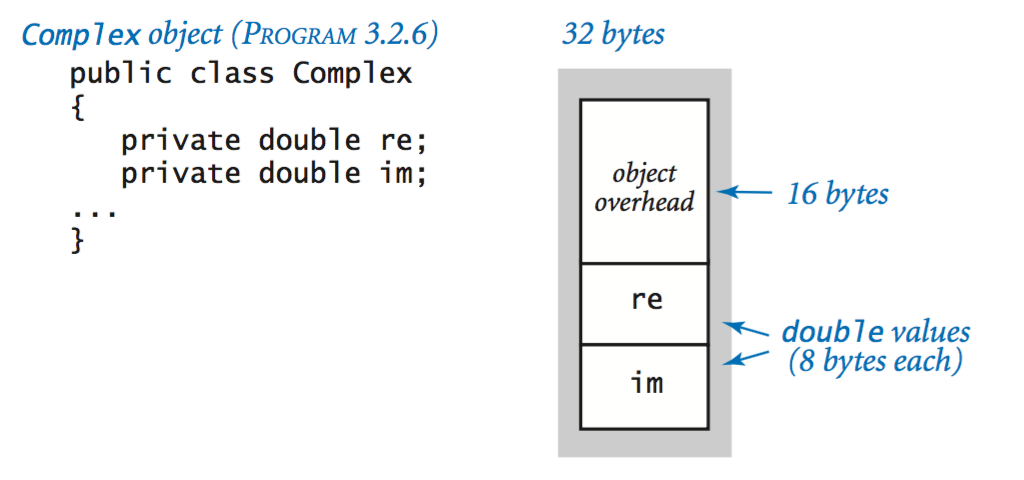A reference to an object typically uses 8 bytes of memory. When a data type contains a reference to an object, we have to account separately for the 8 bytes for the reference and the 16 bytes overhead for each object, plus the memory needed for the object's instance variables.
• Arrays and strings. Arrays in Java are implemented as objects, typically with two instance variables (a pointer to the memory location of the first array element and the length). For primitive types, an array of $$n$$ elements uses 24 bytes of header information, plus $$n$$ times the number of bytes needed to store an element. A two-dimensional array in Java is an array of arrays. For example, an $$n$$-by-$$n$$ array of integers uses $$\sim 4n^2$$ bytes of memory. A string of length $$n$$ uses $$56 + 2n$$ bytes of memory.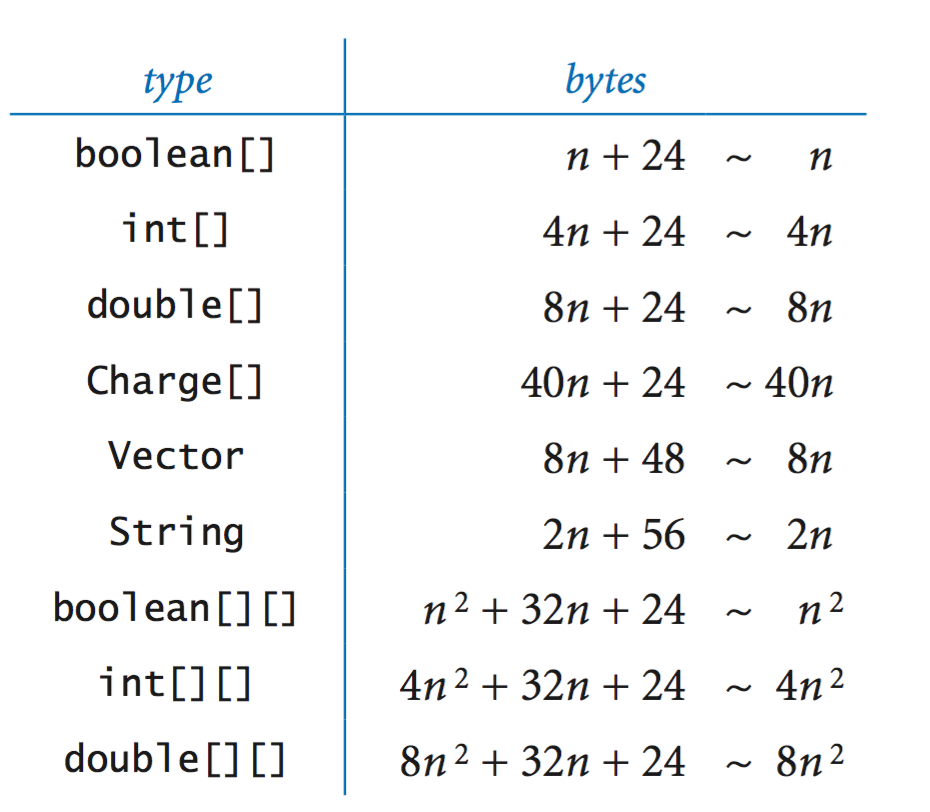See the textbook for full details.

#### Exercises

1. Implement the method printAll() for ThreeSum.java, which prints all of the triples that sum to zero.
2. Write a program FourSum.java that takes an integer reads long integers from standard input, and counts the number of 4-tuples that sum to zero. Use a quadruple nested loop. What is the order of growth of the running time of your program? Estimate the largest input size that your program can handle in an hour. Then, run your program to validate your hypothesis.
3. What is the value of the variable count, as a function of $$n$$, after running the following code fragment?
 int count = 0; for (int i = 0; i < n; i++) for (int j = i+1; j < n; j++) for (int k = j+1; k < n; k++) count++; 
Solution: $$\displaystyle { n \choose 3} = n (n-1) (n-2) / 6$$.
4. Determine the order of growth of the running time of this statement in ThreeSum as a function of the number of integers n on standard input:
 int[] a = StdIn.readAllInts(); 
Solution: Linear. The bottlenecks are the array initialization and the input loop. Depending on your system, however, the cost of an input loop like this might dominate in a linearithmic-time or even a quadratic-time program unless the input size is sufficiently large.
5. Which would you prefer: a quadratic, linearithmic, or linear algorithm?

Solution: While it is tempting to make a quick decision based on the order of growth, it is very easy to be misled by doing so. You need to have some idea of the problem size and of the relative value of the leading coefficients of the running time. For example, suppose that the running times are $$n^2$$ seconds, $$100 n \log_2 n$$ seconds, and $$10000 n$$ seconds. The quadratic algorithm will be fastest for $$n$$ up to about $$1000$$, and the linear algorithm will never be faster than the linearithmic one ($$n$$ would have to be greater than $$2^{100}$$, far too large to bother considering).

6. Apply the scientific method to develop and validate a hypothesis about order of growth of the running time of each of the following two code fragments as a function of $$n$$.
 String s = ""; for (int i = 0; i < n; i++) { if (StdRandom.bernoulli(0.5)) s += "0"; else s += "1"; } StringBuilder sb = new StringBuilder(); for (int i = 0; i < n; i++) { if (StdRandom.bernoulli(0.5)) sb.append("0"); else sb.append("1"); } String s = sb.toString(); 

Solution: The first is quadratic; the second is linear.

7. Give a linear-time algorithm for reversing a string.

Solution:

 public static String reverse(String s) { int n = s.length(); char[] a = new char[n]; for (int i = 0; i < n; i++) a[i] = s.charAt(n-i-1); String reverse = new String(a); return reverse; } 

#### Creative Exercises

1. Subset sum. Write a program SubsetSum.java that reads long integers from standard input, and counts the number of subsets of those integers that sum to exactly zero. Give the order of growth of your algorithm.

2. Sub-exponential function. Find a function whose order of growth is larger than any polynomial function, but smaller than any exponential function. Extra credit: Find a program whose running time has that order of growth.

Solution: $$n^{\ln n}$$.

#### Web Exercises

1. Suppose the running time of an algorithm on inputs of size 1,000, 2,000, 3,000, and 4,000 is 5 seconds, 20 seconds, 45 seconds, and 80 seconds, respectively. Estimate how long it will take to solve a problem of size 5,000. Is the order of growth of the running time of the linear, linearithmic, quadratic, cubic, or exponential?

 for (int i = 0; i < n; i++) for (int j = 0; j < n; j++) for (int k = 0; k < n; k++) c[i][j] += a[i][k] * b[k][j]; 
 public static String random(int n) { if (n == 0) return ""; int r = StdRandom.uniform(26); // between 0 and 25 char c = 'a' + r; // between 'a' and 'z' return random(n/2) + c + random(n - n/2 - 1); }